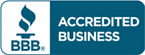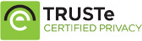# Test bank for Introductory Statistics 9th edition by Neil A. Weiss

\$30.00

Introductory Statistics 9th edition by Neil A. Weiss Test bank Questions

Full Chapters are included

Do you need Solution manual for this book?Categories: ,

## Description

Test bank for Introductory Statistics 9th edition by Neil A. Weiss

Table of Contants

Part I: Introduction
1. The Nature of Statistics
1.1 Statistics Basics
1.2 Simple Random Sampling
1.3 Other Sampling Designs*
1.4 Experimental Designs*
Part II: Descriptive Statistics
2. Organizing Data
2.1 Variables and Data
2.2 Organizing Qualitative Data
2.3 Organizing Quantitative Data
2.4 Distribution Shapes
3. Descriptive Measures
3.1 Measures of Center
3.2 Measures of Variation
3.3 The Five-Number Summary; Boxplots
3.4 Descriptive Measures for Populations; Use of Samples
Part III: Probability, Random Variables, and Sampling Distributions
4. Probability Concepts
4.1 Probability Basics
4.2 Events
4.3 Some Rules of Probability
4.4 Contingency Tables; Joint and Marginal Probabilities*
4.5 Conditional Probability*
4.6 The Multiplication Rule; Independence*
4.7 Bayes’s Rule*
4.8 Counting Rules*
5. Discrete Random Variables*
5.1 Discrete Random Variables and Probability Distributions*
5.2 The Mean and Standard Deviation of a Discrete Random Variable*
5.3 The Binomial Distribution*
5.4 The Poisson Distribution*
6. The Normal Distribution
6.1 Introducing Normally Distributed Variables
6.2 Areas Under the Standard Normal Curve
6.3 Working with Normally Distributed Variables
6.4 Assessing Normality; Normal Probability Plots
6.5 Normal Approximation to the Binomial Distribution*
7. The Sampling Distribution of the Sample Mean
7.1 Sampling Error; the Need for Sampling Distributions
7.2 The Mean and Standard Deviation of the Sample Mean
7.3 The Sampling Distribution of the Sample Mean
Part IV: Inferential Statistics
8. Confidence Intervals for One Population Mean
8.1 Estimating a Population Mean
8.2 Confidence Intervals for One Population Mean When σ Is Known
8.3 Margin of Error
8.4 Confidence Intervals for One Population Mean When σ Is Unknown
9. Hypothesis Tests for One Population Mean
9.1 The Nature of Hypothesis Testing
9.2 Critical-Value Approach to Hypothesis Testing
9.3 P-Value Approach to Hypothesis Testing
9.4 Hypothesis Tests for One Population Mean When σ Is Known
9.5 Hypothesis Tests for One Population Mean When σ Is Unknown
9.6 The Wilcoxon Signed-Rank Test*
9.7 Type II Error Probabilities; Power*
9.8 Which Procedure Should Be Used?*
10. Inferences for Two Population Means
10.1 The Sampling Distribution of the Difference between Two Sample Means for Independent Samples
10.2 Inferences for Two Population Means, Using Independent Samples: Standard Deviations Assumed Equal
10.3 Inferences for Two Population Means, Using Independent Samples: Standard Deviations Not Assumed Equal
10.4 The Mann–Whitney Test*
10.5 Inferences for Two Population Means, Using Paired Samples
10.6 The Paired Wilcoxon Signed-Rank Test*
10.7 Which Procedure Should Be Used?*
11. Inferences for Population Standard Deviations*
11.1 Inferences for One Population Standard Deviation*
11.2 Inferences for Two Population Standard Deviations, Using Independent Samples*
12. Inferences for Population Proportions
12.1 Confidence Intervals for One Population Proportion
12.2 Hypothesis Tests for One Population Proportion
12.3 Inferences for Two Population Proportions
13. Chi-Square Procedures
13.1 The Chi-Square Distribution
13.2 Chi-Square Goodness-of-Fit Test
13.3 Contingency Tables; Association
13.4 Chi-Square Independence Test
13.5 Chi-Square Homogeneity Test
Part V: Regression, Correlation, and ANOVA
14. Descriptive Methods in Regression and Correlation
14.1 Linear Equations with One Independent Variable
14.2 The Regression Equation
14.3 The Coefficient of Determination
14.4 Linear Correlation
15. Inferential Methods in Regression and Correlation
15.1 The Regression Model; Analysis of Residuals
15.2 Inferences for the Slope of the Population Regression Line
15.3 Estimation and Prediction
15.4 Inferences in Correlation
15.5 Testing for Normality*
16. Analysis of Variance (ANOVA)
16.1 The F-Distribution
16.2 One-Way ANOVA: The Logic
16.3 One-Way ANOVA: The Procedure
16.4 Multiple Comparisons*
16.5 The Kruskal–Wallis Test*
Part VI: Multiple Regression and Model Building; Experimental Design and ANOVA (On The WeissStats CD-ROM)
Module A. Multiple Regression Analysis
A.1 The Multiple Linear Regression Model
A.2 Estimation of the Regression Parameters
A.3 Inferences Concerning the Utility of the Regression Model
A.4 Inferences Concerning the Utility of Particular Predictor Variables
A.5 Confidence Intervals for Mean Response; Prediction Intervals for Response
A.6 Checking Model Assumptions and Residual Analysis
Module B. Model Building in Regression
B.1 Transformations to Remedy Model Violations
B.2 Polynomial Regression Model
B.3 Qualitative Predictor Variables
B.4 Multicollinearity
B.5 Model Selection: Stepwise Regression
B.6 Model Selection: All Subsets Regression
B.7 Pitfalls and Warnings
Module C. Design of Experiments and Analysis of Variance
C.1 Factorial Designs
C.2 Two-Way ANOVA: The Logic
C.3 Two-Way ANOVA: The Procedure
C.4 Two-Way ANOVA: Multiple Comparisons
C.5 Randomized Block Designs
C.6 Randomized Block ANOVA: The Logic
C.7 Randomized Block ANOVA: The Procedure
C.8 Randomized Block ANOVA: Multiple Comparisons
C.9 Friedman’s Nonparametric Test for the Randomized Block Design*
APPENDICES
Appendix A: Statistical Tables
I. Random numbers
II. Areas under the standard normal curve
III. Normal scores
IV. Values of tα
V. Values of Wα
VI. Values of Mα
VII. Values of χα2
VIII. Values of Fα
IX. Critical values for a correlation test for normality
X. Values of q0.01
XI. Values of q0.05
XII. Binomial probabilities
Appendix B Answers to Selected Exercises
Index
Photo Credits
Indexes for Biographical Sketches & Case Studies
WeissStats CD-ROM (included with every new textbook)
Brief Contents
Note: See the WeissStats CD ReadMe file for detailed contents.
Applets
Data Sets